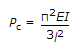# Civil Engineering - Steel Structure Design

### Exercise :: Steel Structure Design - Section 1

41.

The permissible stress in bending for rolled steel I-beams and channels, is

 A. 1500 kg/cm2 B. 1575 kg/cm2 C. 945 kg/cm2 D. 1650 kg/cm2 E. 1875 kg/cm2

Explanation:

No answer description available for this question. Let us discuss.

42.

Column footing is provided

 A. to spread the column load over a larger area B. to ensure that intensity of bearing pressure between the column footing and soil does not exceed permissible bearing capacity of the soil C. to distribute the column load over soil through the column footing D. all the above.

Explanation:

No answer description available for this question. Let us discuss.

43.

Pick up the correct statement from the following:

 A. When the gauge distance is larger than the pitch, the failure of the section may occur in a zig-zag line B. When the gauge distance is smaller than the pitch, the failure of the section may occur in a straight right angle section through the centre of rivet holes C. When the gauge distance and pitch are both equal, the failure to the section becomes more likely as the diameter of the holes increases D. All the above.

Explanation:

No answer description available for this question. Let us discuss.

44.

The ratio of longitudinal stress to strain within eiastic limit, is known as

 A. modulus of elasticity B. shear modulus of elasticity C. bulk modulus of elasticity D. tangent modulus of elasticity E. all the above.

Explanation:

No answer description available for this question. Let us discuss.

45.

If flexural rigidity of a column whose length is L and the loaded end is free, is El, the critical load will be

 A.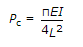B.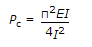C.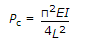D.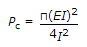E.